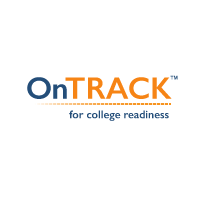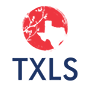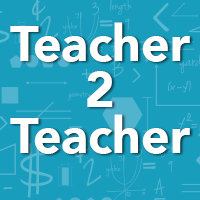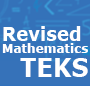# Find resources aligned to Texas Essential Knowledge and Skills (TEKS)

Reset to all
M.6.1
Mathematical process standards. The student uses mathematical processes to acquire and demonstrate mathematical understanding. The student is expected to:
M.6.1.A
apply mathematics to problems arising in everyday life, society, and the workplace;Applying the Order of Operations
Resource ID: M6M1L1 Grade Range: 6 Subject: Math
Given a numerical expression including whole number exponents and prime factorization, students will use the order of operations to generate an equivalent expression.Generating Equivalent Forms of Rational Numbers
Resource ID: M6M1L2 Grade Range: 6 Subject: Math
Given information about a rational number in one form (fraction, decimal, or percent) in a real-world context such as financial mathematics, students will generate an equivalent rational number in a d...2 OnTRACK Grade 6 Math Module 1
This OnTRACK Grade 6 math module feature resources that touch upon student expectations for mathematical process standards, number and operations, proportionality, and personal financial literacy.Perfecting Percents
Lesson Study ID: TXLSR4.001 Grade Range: 6 Subject: Math
Students will engage in an activity that allows them to explore the different parts of percents: part, whole, and percent, and develop conceptual understanding of percents through the Concrete, Repres...
M.6.1.B
use a problem-solving model that incorporates analyzing given information, formulating a plan or strategy, determining a solution, justifying the solution, and evaluating the problem-solving process and the reasonableness of the solution;Applying the Order of Operations
Resource ID: M6M1L1 Grade Range: 6 Subject: Math
Given a numerical expression including whole number exponents and prime factorization, students will use the order of operations to generate an equivalent expression.Generating Equivalent Forms of Rational Numbers
Resource ID: M6M1L2 Grade Range: 6 Subject: Math
Given information about a rational number in one form (fraction, decimal, or percent) in a real-world context such as financial mathematics, students will generate an equivalent rational number in a d...2 OnTRACK Grade 6 Math Module 1
This OnTRACK Grade 6 math module feature resources that touch upon student expectations for mathematical process standards, number and operations, proportionality, and personal financial literacy.Hopping into Real-World Ratios
Lesson Study ID: TXLSR20.006 Grade Range: 6 Subject: Math
Students will listen to a book read aloud called If You Hopped Like a Frog that introduces students to proportions. Students will then create word problems for the different animals and their proporti...Perfecting Percents
Lesson Study ID: TXLSR4.001 Grade Range: 6 Subject: Math
Students will engage in an activity that allows them to explore the different parts of percents: part, whole, and percent, and develop conceptual understanding of percents through the Concrete, Repres...
M.6.1.C
select tools, including real objects, manipulatives, paper and pencil, and technology as appropriate, and techniques, including mental math, estimation, and number sense as appropriate, to solve problems;Perfecting Percents
Lesson Study ID: TXLSR4.001 Grade Range: 6 Subject: Math
Students will engage in an activity that allows them to explore the different parts of percents: part, whole, and percent, and develop conceptual understanding of percents through the Concrete, Repres...
M.6.1.D
communicate mathematical ideas, reasoning, and their implications using multiple representations, including symbols, diagrams, graphs, and language as appropriate;Applying the Order of Operations
Resource ID: M6M1L1 Grade Range: 6 Subject: Math
Given a numerical expression including whole number exponents and prime factorization, students will use the order of operations to generate an equivalent expression.2 OnTRACK Grade 6 Math Module 1
This OnTRACK Grade 6 math module feature resources that touch upon student expectations for mathematical process standards, number and operations, proportionality, and personal financial literacy.
M.6.1.E
create and use representations to organize, record, and communicate mathematical ideas;Generating Equivalent Forms of Rational Numbers
Resource ID: M6M1L2 Grade Range: 6 Subject: Math
Given information about a rational number in one form (fraction, decimal, or percent) in a real-world context such as financial mathematics, students will generate an equivalent rational number in a d...2 OnTRACK Grade 6 Math Module 1
This OnTRACK Grade 6 math module feature resources that touch upon student expectations for mathematical process standards, number and operations, proportionality, and personal financial literacy.
M.6.1.F
analyze mathematical relationships to connect and communicate mathematical ideas; andGenerating Equivalent Forms of Rational Numbers
Resource ID: M6M1L2 Grade Range: 6 Subject: Math
Given information about a rational number in one form (fraction, decimal, or percent) in a real-world context such as financial mathematics, students will generate an equivalent rational number in a d...2 OnTRACK Grade 6 Math Module 1
This OnTRACK Grade 6 math module feature resources that touch upon student expectations for mathematical process standards, number and operations, proportionality, and personal financial literacy.
M.6.1.G
display, explain, and justify mathematical ideas and arguments using precise mathematical language in written or oral communication.Applying the Order of Operations
Resource ID: M6M1L1 Grade Range: 6 Subject: Math
Given a numerical expression including whole number exponents and prime factorization, students will use the order of operations to generate an equivalent expression.2 OnTRACK Grade 6 Math Module 1
This OnTRACK Grade 6 math module feature resources that touch upon student expectations for mathematical process standards, number and operations, proportionality, and personal financial literacy.
M.6.2
Number and operations. The student applies mathematical process standards to represent and use rational numbers in a variety of forms. The student is expected to:
M.6.2.A
classify whole numbers, integers, and rational numbers using a visual representation such as a Venn diagram to describe relationships between sets of numbers;
M.6.2.B
identify a number, its opposite, and its absolute value;
M.6.2.C
locate, compare, and order integers and rational numbers using a number line;
M.6.2.D
order a set of rational numbers arising from mathematical and real-world contexts; and
M.6.2.E
extend representations for division to include fraction notation such as $$a/b$$ represents the same number as $$a ÷ b$$ where $$b \not = 0$$.
M.6.3
Number and operations. The student applies mathematical process standards to represent addition, subtraction, multiplication, and division while solving problems and justifying solutions. The student is expected to:
M.6.3.A
recognize that dividing by a rational number and multiplying by its reciprocal result in equivalent values;Fraction Division
Resource ID: T2T14 Grade Range: 5 - 7 Subject: Math
Explain why the algorithm works.
M.6.3.B
determine, with and without computation, whether a quantity is increased or decreased when multiplied by a fraction, including values greater than or less than one;
M.6.3.C
represent integer operations with concrete models and connect the actions with the models to standardized algorithms;15 Teacher2Teacher Math Video Series
Explore the Teacher2Teacher math video series featuring key topics in mathematics instruction. Bookmark and return to this resource. New videos will be added throughout the year.
M.6.3.D
add, subtract, multiply, and divide integers fluently; andApplying the Order of Operations
Resource ID: M6M1L1 Grade Range: 6 Subject: Math
Given a numerical expression including whole number exponents and prime factorization, students will use the order of operations to generate an equivalent expression.15 Teacher2Teacher Math Video Series
Explore the Teacher2Teacher math video series featuring key topics in mathematics instruction. Bookmark and return to this resource. New videos will be added throughout the year.2 OnTRACK Grade 6 Math Module 1
This OnTRACK Grade 6 math module feature resources that touch upon student expectations for mathematical process standards, number and operations, proportionality, and personal financial literacy.
M.6.3.E
multiply and divide positive rational numbers fluently.Fraction Division
Resource ID: T2T14 Grade Range: 5 - 7 Subject: Math
Explain why the algorithm works.How Does the Cookie Crumble?
Lesson Study ID: TXLS031 Grade Range: 6 Subject: Math
Students will self-discover how to multiply mixed numbers by using background knowledge of estimation, computations, and real world application of a recipe.   Texas Ess...
M.6.4
Proportionality. The student applies mathematical process standards to develop an understanding of proportional relationships in problem situations. The student is expected to:
M.6.4.A
compare two rules verbally, numerically, graphically, and symbolically in the form of $$y = ax$$ or$y = x + a$ in order to differentiate between additive and multiplicative relationships;
M.6.4.B
apply qualitative and quantitative reasoning to solve prediction and comparison of real-world problems involving ratios and rates;Hopping into Real-World Ratios
Lesson Study ID: TXLSR20.006 Grade Range: 6 Subject: Math
Students will listen to a book read aloud called If You Hopped Like a Frog that introduces students to proportions. Students will then create word problems for the different animals and their proporti...
M.6.4.C
give examples of ratios as multiplicative comparisons of two quantities describing the same attribute;
M.6.4.D
give examples of rates as the comparison by division of two quantities having different attributes, including rates as quotients;
M.6.4.E
represent ratios and percents with concrete models, fractions, and decimals;
M.6.4.F
represent benchmark fractions and percents such as 1%, 10%, 25%, 33 1/3%, and multiples of these values using 10 by 10 grids, strip diagrams, number lines, and numbers;
M.6.4.G
generate equivalent forms of fractions, decimals, and percents using real-world problems, including problems that involve money; andGenerating Equivalent Forms of Rational Numbers
Resource ID: M6M1L2 Grade Range: 6 Subject: Math
Given information about a rational number in one form (fraction, decimal, or percent) in a real-world context such as financial mathematics, students will generate an equivalent rational number in a d...2 OnTRACK Grade 6 Math Module 1
This OnTRACK Grade 6 math module feature resources that touch upon student expectations for mathematical process standards, number and operations, proportionality, and personal financial literacy.
M.6.4.H
convert units within a measurement system, including the use of proportions and unit rates.15 Teacher2Teacher Math Video Series
Grade Range: 2 - 5 Subject: Math
Explore the Teacher2Teacher math video series featuring key topics in mathematics instruction. Bookmark and return to this resource. New videos will be added throughout the year.
M.6.5
Proportionality. The student applies mathematical process standards to solve problems involving proportional relationships. The student is expected to:
M.6.5.A
represent mathematical and real-world problems involving ratios and rates using scale factors, tables, graphs, and proportions;Roll With It
Lesson Study ID: TXLS066 Grade Range: 6 Subject: Math
Students will experience a hands-on lesson regarding ratios. While doing this, students will deepen their understanding of the concepts of ratios. Teacher during Introd...
M.6.5.B
solve real-world problems to find the whole given a part and the percent, to find the part given the whole and the percent, and to find the percent given the part and the whole, including the use of concrete and pictorial models; andPerfecting Percents
Lesson Study ID: TXLSR4.001 Grade Range: 6 Subject: Math
Students will engage in an activity that allows them to explore the different parts of percents: part, whole, and percent, and develop conceptual understanding of percents through the Concrete, Repres...Perfectly Proportional Percents
Lesson Study ID: TXLSR10.018 Grade Range: 6 Subject: Math
Students will collaborate to explain verbally how to solve percent proportions and scaling while showing their thinking.
M.6.5.C
use equivalent fractions, decimals, and percents to show equal parts of the same whole.Generating Equivalent Forms of Rational Numbers
Resource ID: M6M1L2 Grade Range: 6 Subject: Math
Given information about a rational number in one form (fraction, decimal, or percent) in a real-world context such as financial mathematics, students will generate an equivalent rational number in a d...2 OnTRACK Grade 6 Math Module 1
This OnTRACK Grade 6 math module feature resources that touch upon student expectations for mathematical process standards, number and operations, proportionality, and personal financial literacy.
M.6.6
Expressions, equations, and relationships. The student applies mathematical process standards to use multiple representations to describe algebraic relationships. The student is expected to:
M.6.6.A
identify independent and dependent quantities from tables and graphs;Independent and Dependent Variable in Tables and Graphs
Lesson Study ID: TXLSR14.020 Grade Range: 6 Subject: Math
Students will use information in a real-world scenario to create a table or graph, translate the meaning of the table or graph, and identify the independent and dependent quantities. ...
M.6.6.B
write an equation that represents the relationship between independent and dependent quantities from a table; andMultiple Representations: Tables to Equations and Graphs
Resource ID: T2T10 Grade Range: 6 - 8 Subject: Math
Learn about differences between multiple representations in 8th grade and in Algebra I, including tables, equations, and graphs.15 Teacher2Teacher Math Video Series
Grade Range: 6 - 8 Subject: Math
Explore the Teacher2Teacher math video series featuring key topics in mathematics instruction. Bookmark and return to this resource. New videos will be added throughout the year.
M.6.6.C
represent a given situation using verbal descriptions, tables, graphs, and equations in the form $$y = kx$$ or $$y = x + b$$.Multiple Representations: Tables to Equations and Graphs
Resource ID: T2T10 Grade Range: 6 - 8 Subject: Math
Learn about differences between multiple representations in 8th grade and in Algebra I, including tables, equations, and graphs.Multiple Representations: Equations to Tables and Graphs
Resource ID: T2T11 Grade Range: 6 - 8 Subject: Math
Multiple Representations: Equations to Tables and Graphs15 Teacher2Teacher Math Video Series
Grade Range: 6 - 8 Subject: Math
Explore the Teacher2Teacher math video series featuring key topics in mathematics instruction. Bookmark and return to this resource. New videos will be added throughout the year.
M.6.7
Expressions, equations, and relationships. The student applies mathematical process standards to develop concepts of expressions and equations. The student is expected to:Express Yourself
Lesson Study ID: TXLSR11.026 Grade Range: 6 Subject: Math
Students determine which expression is a truth or a lie by generating equivalent expressions.
M.6.7.A
generate equivalent numerical expressions using order of operations, including whole number exponents and prime factorization;Applying the Order of Operations
Resource ID: M6M1L1 Grade Range: 6 Subject: Math
Given a numerical expression including whole number exponents and prime factorization, students will use the order of operations to generate an equivalent expression.2 OnTRACK Grade 6 Math Module 1
This OnTRACK Grade 6 math module feature resources that touch upon student expectations for mathematical process standards, number and operations, proportionality, and personal financial literacy.Express Yourself
Lesson Study ID: TXLSR11.026 Grade Range: 6 Subject: Math
Students determine which expression is a truth or a lie by generating equivalent expressions.
M.6.7.B
distinguish between expressions and equations verbally, numerically, and algebraically;
M.6.7.C
determine if two expressions are equivalent using concrete models, pictorial models, and algebraic representations; and15 Teacher2Teacher Math Video Series
Grade Range: 6 - 8 Subject: Math
Explore the Teacher2Teacher math video series featuring key topics in mathematics instruction. Bookmark and return to this resource. New videos will be added throughout the year.
M.6.7.D
generate equivalent expressions using the properties of operations: inverse, identity, commutative, associative, and distributive properties.15 Teacher2Teacher Math Video Series
Grade Range: 6 - 8 Subject: Math
Explore the Teacher2Teacher math video series featuring key topics in mathematics instruction. Bookmark and return to this resource. New videos will be added throughout the year.
M.6.8
Expressions, equations, and relationships. The student applies mathematical process standards to use geometry to represent relationships and solve problems. The student is expected to:
M.6.8.A
extend previous knowledge of triangles and their properties to include the sum of angles of a triangle, the relationship between the lengths of sides and measures of angles in a triangle, and determining when three lengths form a triangle;
M.6.8.B
model area formulas for parallelograms, trapezoids, and triangles by decomposing and rearranging parts of these shapes;Area of Triangles, Parallelograms, and Trapezoids
Resource ID: TEKS12_MATH_06_002 Grade Range: 6 Subject: Math
These activities provide an opportunity for students to explore the area formulas for triangles, trapezoids, and parallelograms.
M.6.8.C
write equations that represent problems related to the area of rectangles, parallelograms, trapezoids, and triangles and volume of right rectangular prisms where dimensions are positive rational numbers; and
M.6.8.D
determine solutions for problems involving the area of rectangles, parallelograms, trapezoids, and triangles and volume of right rectangular prisms where dimensions are positive rational numbers.
M.6.9
Expressions, equations, and relationships. The student applies mathematical process standards to use equations and inequalities to represent situations. The student is expected to:
M.6.9.A
write one-variable, one-step equations and inequalities to represent constraints or conditions within problems;Multiple Representations: Verbal to Equations
Resource ID: T2T12 Grade Range: 5 - 9 Subject: Math
Multiple Representations: Verbal to Equations15 Teacher2Teacher Math Video Series
Grade Range: 5 - 9 Subject: Math
Explore the Teacher2Teacher math video series featuring key topics in mathematics instruction. Bookmark and return to this resource. New videos will be added throughout the year.
M.6.9.B
represent solutions for one-variable, one-step equations and inequalities on number lines; and